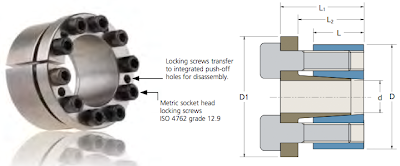## Posts

Showing posts from April, 2012

### Improve math skills of your kids - Learn step-by-step arithmetic from Math games

Math: Unknown - Step-by-step math calculation game for iOS.

Math: Unknown is much more than a math game. It is a step-by-step math calculation game which will teach users how to calculate in the correct order rather than just asking only the final calculated results.

The app consists of four basic arithmetic operations which are addition, subtraction, multiplication and division. In order to get started, users who are new to arithmetic can learn from animated calculation guides showing step-by-step procedures of solving each type of operation. It is also helpful for experienced users as a quick reference.

Generally, addition and subtraction may be difficult for users who just start learning math especially when questions require carrying or borrowing (also called regrouping). The app helps users to visualize the process of carrying and borrowing in the way it will be done on paper. Once users understand how these operations work, they are ready to learn multiplication and division.

For most students, division is considered as the most difficult arithmetic operation to solve. It is a common area of struggle since it requires prior knowledge of both multiplication and subtraction. To help users understand division, the app uses long division to teach all calculation procedures. Relevant multiplication table will be shown beside the question. Users will have to pick a number from the table which go into the dividend. Multiplication of selected number and divisor is automatically calculated, but the users have to do subtraction and drop down the next digit themselves. Learning whole calculation processes will make them master it in no time.

Math: Unknown is a helpful app for students who seriously want to improve arithmetic calculation skills.

### Keyless Bushings for power transmissionThere are several methods to connect shaft and hub together for power transmission. Let's find the advantage of using keyless bushings from Fenner compared with other traditional connection methods.
Traditional Connection MethodsInterference Fits (Shrink and Press)
A shrink fit is a procedure whereby heat is used to facilitate a mechanical interference fit between two pieces of metal, such as a steel shaft and hub. Extreme heat is applied to the hub, causing it to expand and increasing the size of its machined bore. The expanded hub is removed from the heat source and quickly positioned onto the shaft. As the hub cools, its bore contracts back to its original machined dimension, effectively “shrinking” the hub onto the shaft.

A press fit achieves the same end as a shrink fit — a mechanical interference fit between a steel shaft and hub — but does so through different means. Press fits rely on the application of simple brute force to “press” the hub onto the shaft. Interference…